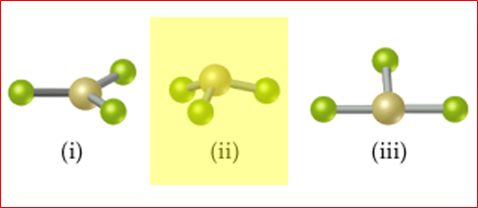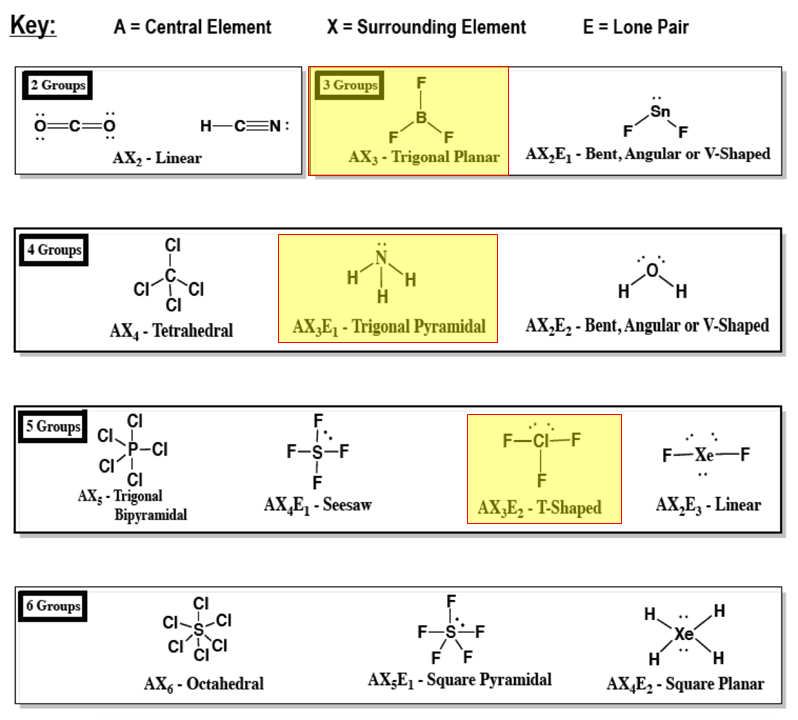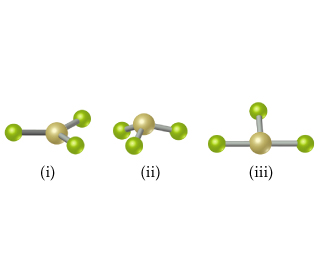# Problem: This figure shows ball-and-stick drawings of three possible shapes of an AF3 molecule. Which of the following elements will lead to an AF3 molecule with the shape in (ii): Li, B, N, Al, P, Cl?

###### FREE Expert Solution

We’re asked to determine which of the following elementsLi, B, N, Al, P, and Cl will lead to an AF3 molecule with the shape in (ii) as shown below:To do so, we first need to draw a Lewis structure for a molecule AFwhere A = central atom, F = terminal atoms for each given element

For this, we need to do the following steps:

Step 1: Assign elements to form a bond and Determine the central atom in the resulting molecule.

Step 2: Calculate the total number of valence electrons present.

Step 3: Draw the Lewis structure for the molecule.

Step 4: Determine the shape of the molecule and whether it matches the shape in (ii):

The possible molecular shapes are:Note that the shapes that follow an AF(or AX3) molecule are: trigonal planar, trigonal pyramidal and T-shaped.

From the given ball and stick figures, we can see that:

79% (27 ratings)###### Problem Details

This figure shows ball-and-stick drawings of three possible shapes of an AF3 molecule.Which of the following elements will lead to an AF3 molecule with the shape in (ii): Li, B, N, Al, P, Cl?